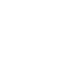# Question of the Day: SAT & ACT May 22

If you are reading this in an email you received from me, do not click the link to sat.collegeboard.org below. Use the link to my website that is farther down on the email. If you are seeing this in my blog, do the SAT Question of the Day by clicking on this link:

http://sat.collegeboard.org/practice/sat-question-of-the-day?src=R&questionId=20130522 (This link takes you to today’s question. If you use my archive, you will see the question related to my SAT explanation for that date.)

The answer is B.  The test writer’s explanation is a good “math teacher” approach to the question and you should take a look at it.  I did it a different way which seemed easier for me.  That’s the thing — there are always multiple ways to do the math questions on the SAT and ACT.  You need to practice so that you can figure out what the best ways are for you.  So much for my advice, let me show you what I did with this question.

Many of the fraction questions are simple proportions that you can do in your head.  Compare the numerators with one another and the denominators with one another.  24 is 6 times as big as 4; therefore, 15 has to be 6 times as big as n.  So, n=15/6=2.5.  That makes 4n=4 times 2.5=10.  If that explanation was easy for you to follow, then you could do many fraction/proportion questions the same way and save some time on the test.  If you like the test writer’s explanation, you should do it that way.

Let’s see what we can do to outsmart the ACT folks.

http://www.act.org/qotd/ (The ACT staff does not put a date on their questions so if you click on an archived blog, you’ll get today’s question and the old explanation. Sorry. The SAT staff has dated their questions; so, the archive is helpful. The ACT folks simply don’t do that.)

This is good.  I’m already in “math” mode.

The answer is E.  This question is a case of just manipulating a simple equation.  To isolate x, we have to get rid of the -5.  Just put it over on the other side of the equal sign and change the sign to +.   Then to get rid of the 2 in 2x, just move the 2 to the other side of the equal sign and divide.  The answer is E.

Have you ever noticed what is happening when you solve algebra equations?  Anytime you perform a math operation to both sides of the equation, it is the same as just moving the variable/number to the other side of the equation and changing the sign.  For example, in this equation if you add 5 to both sides to cancel the -5, you get +5 on the left side.  I think it is quicker to just think about it as moving the 5 to the other side and reversing the math operation — change the -5 to a +5.  To get rid of the 2 in the 2x, just move the 2 to the other side and divide by 2.  With a little practice, you’re algebra skills will speed up.

Have a “Wizardly” day.

Wizard